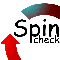# Spinroot

A forum for Spin users

You are not logged in.

## #1 2015-02-02 03:02:50

Dharma
Member
Registered: 2011-09-21
Posts: 13

### Unclear why an assert failed (modex)

The following C code (based on a OS book) implements a concurrent queue. http://pages.cs.wisc.edu/~remzi/OSTEP/threads-locks-usage.pdf (Figure 29.8) has the basic code used here.

In the test driver, I just have one reader and one writer.

I'm not sure why the assert(tmp != NULL) in the Queue_Init false. It happens only if I include the sample_reader as part of the extraction in the prx file.

I'm using the Queue_Init only from Queue_Enqueue, which sets the ready flag to true, making it possible for the Queue_Dequeue to run.

#include <assert.h>

struct __node_t {
int value;
struct __node_t *next;
};

typedef struct __node_t node_t;

struct __queue_t {
node_t *tail;
};

typedef struct __queue_t queue_t;

void Queue_Init(queue_t *q) {
node_t *tmp;

tmp = (node_t*)cq_malloc(sizeof(node_t));
assert(tmp != NULL);
tmp->next = NULL;
}

void Queue_Enqueue(queue_t *q, int value) {
node_t *tmp;

tmp = (node_t*)cq_malloc(sizeof(node_t));
assert(tmp != NULL);
tmp->value = value;
tmp->next = NULL;

q->tail->next = tmp;
q->tail = tmp;
}

int Queue_Dequeue(queue_t *q, int *value) {
node_t *tmp;

return -1; // queue was empty
}

cq_free(tmp);
return 0;
}

/* Test driver */
queue_t queue;

void
sample_writer(void)
{       int i;

Queue_Init(&queue);

for(i = 0; i < 5; i++)
{
Queue_Enqueue(&queue, i);
}

}

void
{
int j = 0;
int i = 0;
int status = -1;

{
;
}

for(i = 0 ; i < 5; i++) {
status = Queue_Dequeue(&queue, &j);
if(status == -1)
{
i = i - 1;
}
else {
assert(i == j);
}
}
}

----

%X -a sample_writer
%X -e Queue_Init
%X -e Queue_Enqueue
%X -e Queue_Dequeue
%G
shortest: 1
%%
%C

#define MAX_HEAP        200

int cnt = 0;
char heap[MAX_HEAP];

char *
cq_malloc(unsigned int n)
{       char *p;
p = (void *) 0;

if (cnt + n <= sizeof(heap))
{       p = &heap[cnt];
cnt += n;
}
return p;
}

void
cq_free(void *p)
{
p = NULL;
}

------------------

Last edited by Dharma (2015-02-02 03:12:26)

Offline

## #2 2015-02-05 18:18:36

spinroot
forumRegistered: 2010-11-18
Posts: 691
Website

### Re: Unclear why an assert failed (modex)

the reason:  cq_malloc returns NULL because the heap you declared in the .prx file is exhausted (it has just 200 bytes).

in the error trail I see a total of six calls to cq_malloc, each allocating the size of a node_t structure, which is 128 bytes on my machine (two times a 64 bit quantity), which exceeds 200 bytes by a fair margin.

[email protected]:~/Dharma\$ spin -replay model| grep cq_malloc
10:    proc  2 (p_Queue_Init) model:137 (state 8)      [ Pp_Queue_Init->tmp=(node_t *)cq_malloc(sizeof(node_t )); ]
41:    proc  1 (p_Queue_Enqueue) model:115 (state 9)   [ Pp_Queue_Enqueue->tmp=(node_t *)cq_malloc(sizeof(node_t )); ]
67:    proc  1 (p_Queue_Enqueue) model:115 (state 9)   [ Pp_Queue_Enqueue->tmp=(node_t *)cq_malloc(sizeof(node_t )); ]
93:    proc  1 (p_Queue_Enqueue) model:115 (state 9)   [ Pp_Queue_Enqueue->tmp=(node_t *)cq_malloc(sizeof(node_t )); ]
119:    proc  1 (p_Queue_Enqueue) model:115 (state 9)   [ Pp_Queue_Enqueue->tmp=(node_t *)cq_malloc(sizeof(node_t )); ]
162:    proc  1 (p_Queue_Enqueue) model:115 (state 9)   [ Pp_Queue_Enqueue->tmp=(node_t *)cq_malloc(sizeof(node_t )); ]

Offline

## #3 2015-02-05 21:09:59

spinroot
forumRegistered: 2010-11-18
Posts: 691
Website

### Re: Unclear why an assert failed (modex)

By the way:  the heap would also need to be visible to Spin to track.
You can do that with a c_track statement, but perhaps it's much easier to just let Modex handle it by using a standard call to malloc() instead.  If you do so, Modex will convert it into a call to an already instrumented internal function called spin_malloc(), which takes care of the tracking.
You can set the size of the internal spin_heap with an argument to modex itself:
modex -heapsize=200
(the default heapsize used by spin/modex is 256 bytes)

Offline